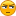# Linear Regression Indicator - page 4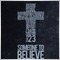2449

As the indicator draws the line, you imagine a continuation of that line, so that where the candle should get after sometime, so you check whats the main suport and resistence, sell/buy on them and use the indicator as a profit target, it's made for side markets.[Deleted]

The LinearRegression-REAL.mq4 is the closest to the plots I get on other platforms but it was not exactly the same. I did some research and found some problems in the formula. I used the info for Excel from here

Excel Tutorial on Linear Regression

to fix the formula.

Two problem I found:

1. In the calculation for Y intercept a + was used and should have been a -

2. The Y plot was being multiplied by the period for some odd reason.

I also cleaned up the code a bit. I now looks much closer to the plots on other platforms. Pics attached. (Note: Candle data is different so curves are not exact but very close.)

Files:127

Petor

newdigital:
It works. I just attach indicator to the chart (MT4) and it works (see image).

Wonderful Regression + MA Chanel is that! Thank You!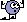23

forecast and intercept

hi guys ,i'm new for this forum , my name is maco castoldi and i'm italian,i'm honored to be here, so, i'm looking for two indicators mt4 : linear regression forecast and linear regrssion intercept.thanks for your help.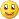55

Anyone trading multiple time frames with Linear Regression channel indicator?

Hello!

I have downloaded the Regression Channel indicator and would like to have a multiple time frame setup where I for example use the Daily, 1H, 15min and 5M with the Regression Channel indicator on each time frame to make a solid direction framework with the indicator.

I am wondering if there are someone who does something like this and have found some good settings with the indicator for this kind of setup?

Thanks,

Laurus1754

are you using statistics to trade

there are MTF thread, you can find lot of MTF indicator, i.e. watch another TF in current tf (TF and tf is diff)

not sure about your regression indic, but you can make enhancement in the coding, like what I did here, -- easier to trade this way, so I keep it for my own use only

Files:49

xx3xxx:
are you using statistics to trade

there are MTF thread, you can find lot of MTF indicator, i.e. watch another TF in current tf (TF and tf is diff)

not sure about your regression indic, but you can make enhancement in the coding, like what I did here, -- easier to trade this way, so I keep it for my own use only

That's nice of you. Following in the community spirit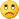9550

That's nice of you. Following in the community spiritDon

Got this one its mtf and multicolor its the same as the one posted by Tigertron few posts above just added mtf,multicolors, and the ability to change the applied price(high,low,etc.) On the chart picture using 80 period with high,low price.

Files:201180

Only yesterday (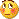) yaw this post and, naturally it drew my attention :

Cases when there is a common msbelief in TA are not that uncommon and was wondering if it is the case with linear regression calculations that I have seen so far as of compared to this one.

Well there is no one more misbelief case. The indicator posted has an error in calculation. By definition y intercept is : "the point where the line crosses the y-axis, in other words, the value of y when x equals 0.". Hence the line containing this code :
LRLBuffer[shift] = slope*1+yint;

is wrong. Multiplier of slope can not be 1 (one), it must be 0. If it is replaced by 0 then you will get a perfect linear regression value, otherwise you are getting a penultimate point on linear regression line (it is not a linear regression value of previous bar, but exactly that : a point 1 bar back on a current linear regression line). So keep using the linear regression indicators, lsma indicators (linear regression value renamed) as before They are correct ...

Tigertron:
The LinearRegression-REAL.mq4 is the closest to the plots I get on other platforms but it was not exactly the same. I did some research and found some problems in the formula. I used the info for Excel from here

Excel Tutorial on Linear Regression

to fix the formula.

Two problem I found:

1. In the calculation for Y intercept a + was used and should have been a -

2. The Y plot was being multiplied by the period for some odd reason.

I also cleaned up the code a bit. I now looks much closer to the plots on other platforms. Pics attached. (Note: Candle data is different so curves are not exact but very close.)9550

Repaired it now the hard part is this version real real, real2,lsma,hmm maybe realernah guess will call it what it was or is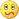. And compared with Lsma was posted yesterday, my question now is, was it first Lsma or Linear Regression